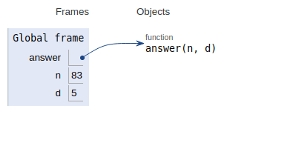# Python Program for Smallest K digit number divisible by X

In this article, we will learn about the solution and approach to solve the given problem statement.

## Problem statement

Integers n and d are given. We need to find the smallest n-digit number divisible by d.

## Approach

1. FirstNow let's we compute MIN : smallest n-digit number (1000...n-times)

2. Now, If MIN % X is 0, ans = MIN

3. else, ans = (MIN + X) - ((MIN + X) % X))

This is because there will be a number in range [MIN...MIN+X] which is divisible by d.

Now let’s see the implementation −

## Example

Live Demo

def answer(n, d):
# Computing MAX
Min = pow(10, d-1)
if(Min%n == 0):
return (Min)
else:
return ((Min + n) - ((Min + n) % n))
n = 83
d = 5
print(answer(n, d))

## Output

10043

All the variables are declared in the global frame as shown in the figure given below −## Conclusion

In this article, we learnt about the approach to find Smallest K digit number divisible by X